// This prints the left floatting menu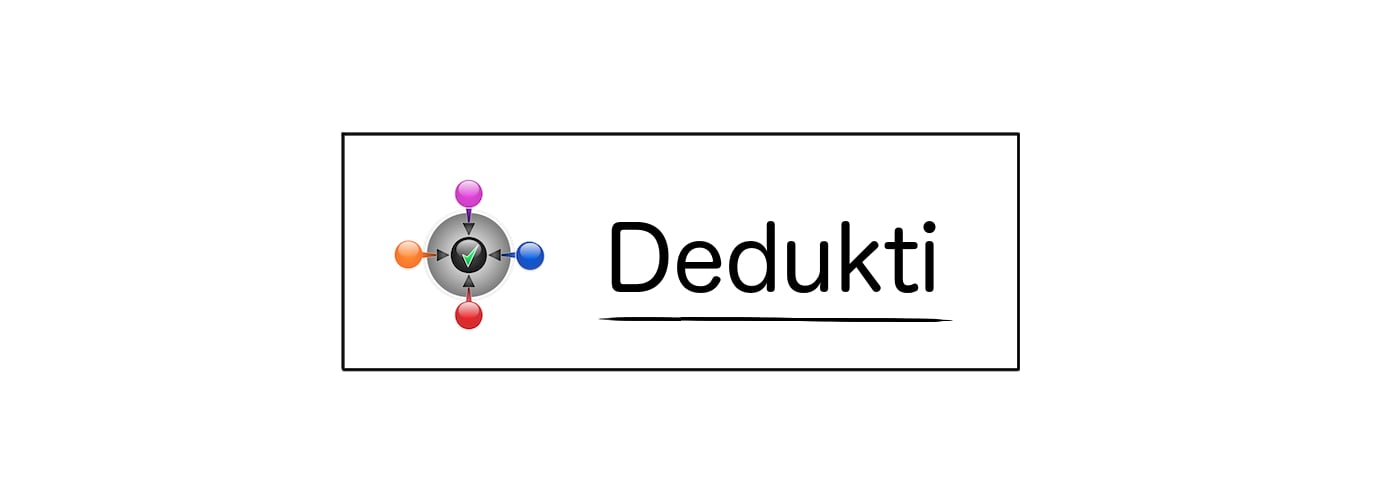### Definitionrelations.reflexive

Body

λR. ∀ x, R x x

Main Dependencies
Theory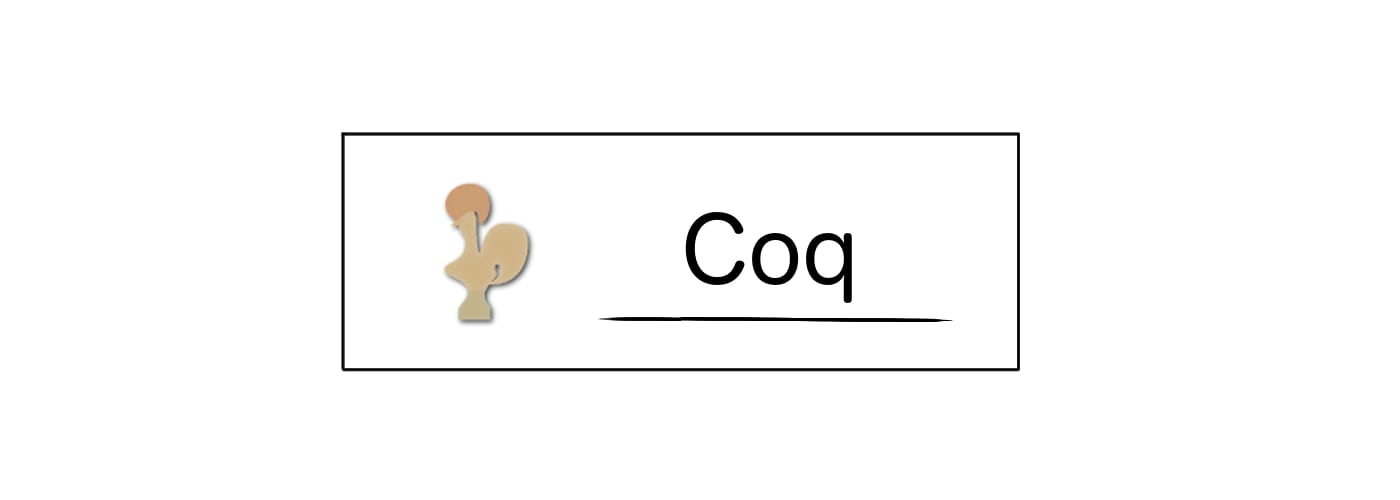Body

Definition reflexive : forall (A:Type), (A -> A -> Prop) -> Prop := fun (A:Type) => fun (R:(A -> A -> Prop)) => forall (x:A), R x x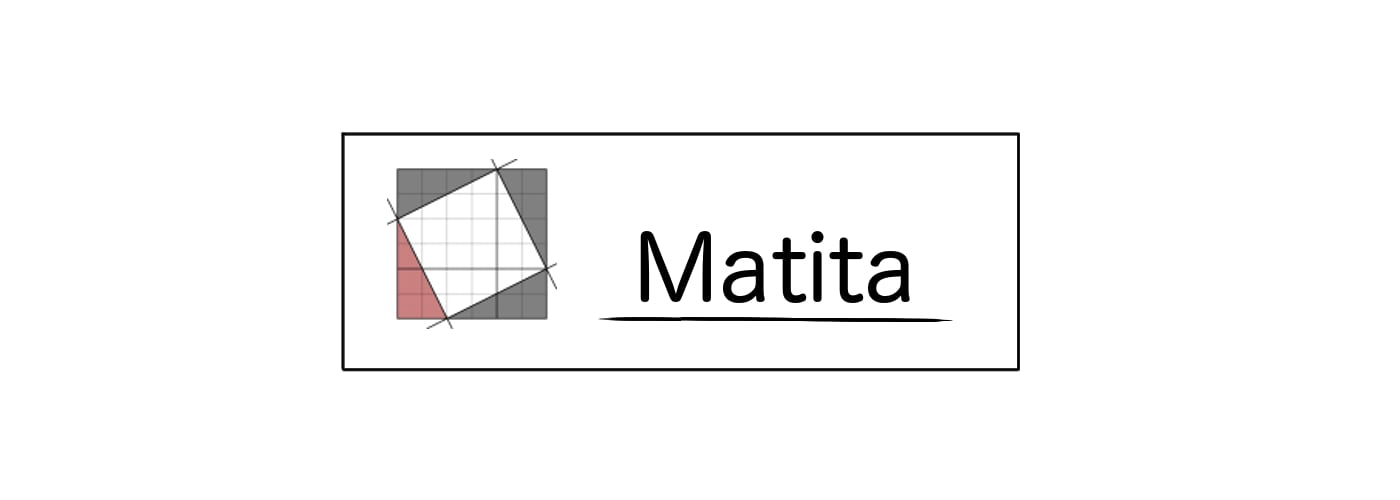Body

definition reflexive : \forall A : Type . (A -> A -> Prop) -> Prop := \lambda A : Type. \lambda R : A -> A -> Prop. \forall (x:A). R x x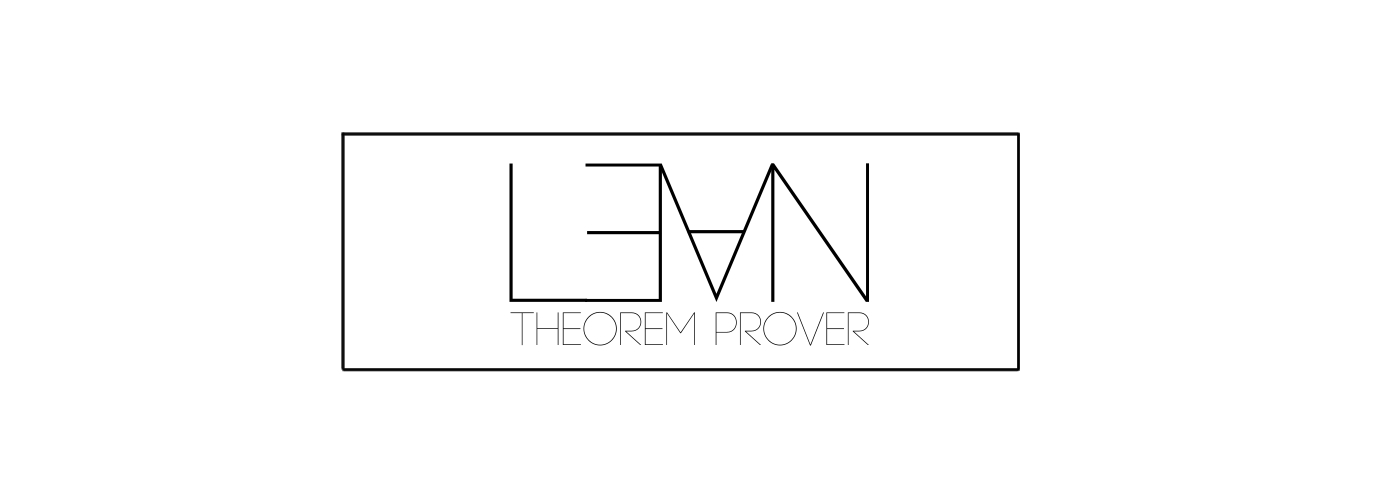Body

def reflexive : forall (A : Type) , ((A) -> (A) -> Prop) -> Prop := fun (A : Type) , fun (R : (A) -> (A) -> Prop) , forall (x:A) , ((R) (x)) (x)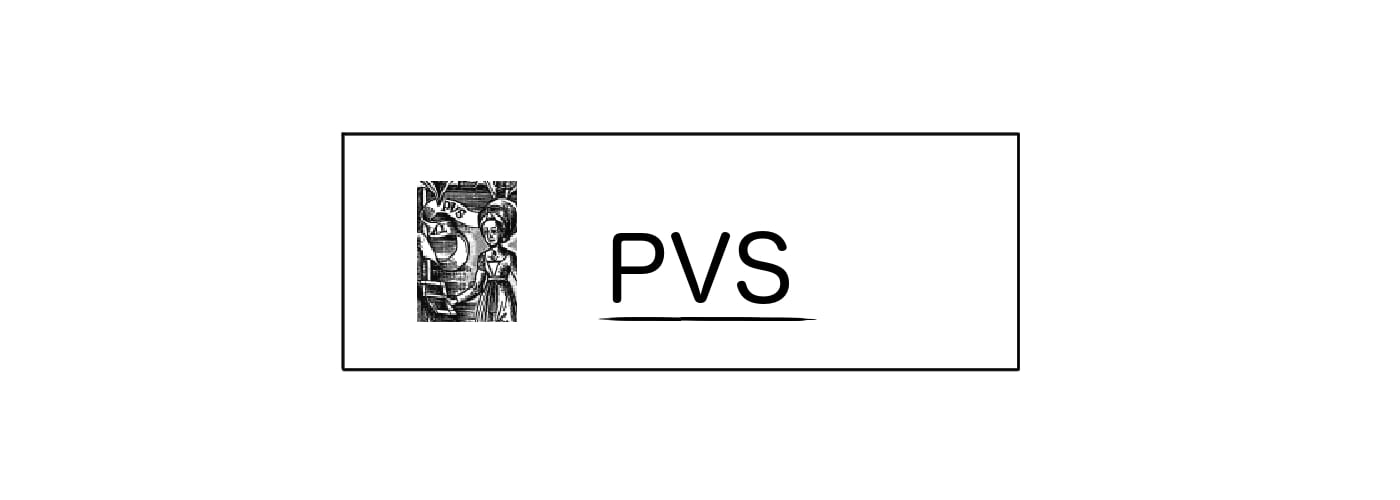Body

reflexive [A:TYPE+] : [[A -> [A -> bool]] -> bool] = (LAMBDA(R:[A -> [A -> bool]]):(FORALL(x:A):R(x)(x)))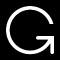Printing for OpenTheory is not working at the moment.